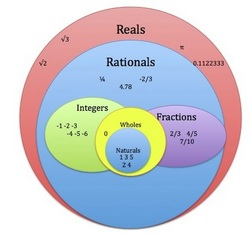# MTH321: Real Analysis 1 (Spring 2015)At the end of this course the students will be able to uunderstand the basic set theoretic statements and emphasize the proofs’ development of various statements by induction. Define the limit of, a function at a value, a sequence and the Cauchy criterion. Prove various theorems about limits of sequences and functions and emphasize the proofs’ development. Define continuity of a function and uniform continuity of a function, prove various theorems about continuous functions and emphasize the proofs’ development. Define the derivative of a function, prove various theorems about the derivatives of functions and emphasize the proofs’ development. Define a cluster point and an accumulation point, prove the Bolzano-Weierstrass theorem, Rolles’s Theorem, extreme value theorem, and the Mean Value theorem and emphasize the proofs’ development. Define Riemann integral and Riemann sums, prove various theorems about Riemann sums and Riemann integrals and emphasize the proofs’ development.

The Real Number System: Ordered Fields. The Field of Reals. The Extended Real Number System. Euclidean Space. Numerical Sequences and Series. Limit of a Sequence. Bounded Sequences. Monotone Sequences. Limits Superior and Inferior. Subsequences. Limit of a Function and Continuous Functions. Uniform Continuity. Kinds of Discontinuities. Derivable and Differentiable Functions. Mean Value theorems. The Continuity of Derivatives. Taylor's theorem. Riemann Stieltijes Integrals: Definition, Existence and Properties of the Riemann Integrals. Integral and Differentiation.

Did you know?

• The development of calculus in the 18th century used the entire set of real numbers without having defined them cleanly. The first rigorous definition was given by Georg Cantor in 1871.
• In the 16th century, Simon Stevin created the basis for modern decimal notation, and insisted that there is no difference between rational and irrational numbers in this regard.

Please download PDF files of the notes handout given below. These files can be only viewed or print if there is PDF reader or viewer installed on your system. See Software section for some PDF reader or viewer.

1. Rudin, W. (1976). Principle of Mathematical Analysis, McGraw Hills Inc.
2. Bartle, R.G., and D.R. Sherbert, (2011): Introduction to Real Analysis, 4th Edition, John Wiley & Sons, Inc.
3. Apostol, Tom M. (1974), Mathematical Analysis, Pearson; 2nd edition.
• atiq/sp15-mth321